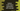# Python math fabs() method explanation with example## Python math fabs method explanation with example:

The fabs method of math module returns the absolute value of a number in Python. This method is defined in the math module and we have to import this module to use this method.

In this post, we will learn how to use the fabs method with examples.

### Syntax of fabs:

The syntax of fabs() method is:

``math.fabs(n)``

This method is defined in the math module. We have to import this module to use it.

### Parameter and return value:

This method takes only one parameter, i.e. the number to find the absolute value. It returns the absolute value of the number n.

### Example of fabs:

Let’s take an example of fabs with different numbers:

``````import math

print(math.fabs(10.23))
print(math.fabs(-.23))
print(math.fabs(-33.45))
print(math.fabs(122/2))``````

It will print the below output:

``````10.23
0.23
33.45
61.0``````

As you can see in this example,

### Example of fabs with user input values:

Let me show you another example of fabs with user-input numbers:

``````from math import fabs

num = float(input("Enter a number: "))
print("Absolute value: {}".format(fabs(num)))``````

This method takes a number as input from the user. It converts that number to float and stores that in the variable num. The last line is using fabs to print the absolute value of the number.

It will give output as like below:

``````Enter a number: 12.34
Absolute value: 12.34

Enter a number: -998.28
Absolute value: 998.28``````